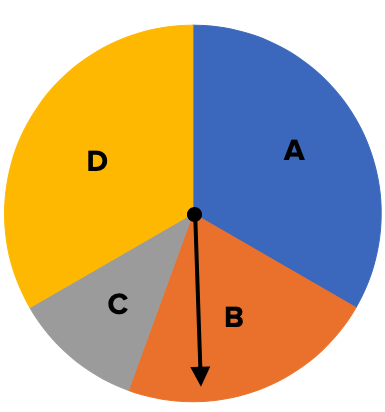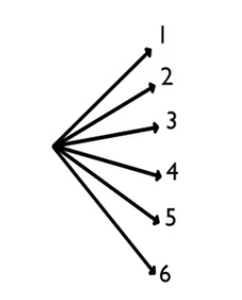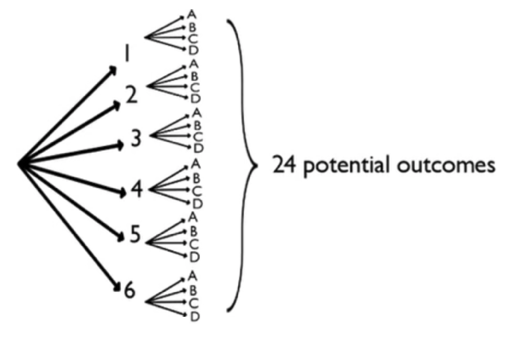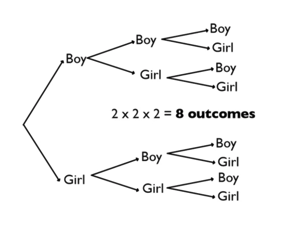+
Common Core: 7.SP.8b

# Fundamental Counting Principle

Author: Sophia Tutorial
##### Description:

Calculate the number of possible outcomes for a given situation using the fundamental counting principle.

(more)

Sophia’s self-paced online courses are a great way to save time and money as you earn credits eligible for transfer to many different colleges and universities.*

No credit card required

37 Sophia partners guarantee credit transfer.

299 Institutions have accepted or given pre-approval for credit transfer.

* The American Council on Education's College Credit Recommendation Service (ACE Credit®) has evaluated and recommended college credit for 32 of Sophia’s online courses. Many different colleges and universities consider ACE CREDIT recommendations in determining the applicability to their course and degree programs.

Tutorial
what's covered
This tutorial will explain the fundamental counting principle. Our discussion breaks down as follows:

1. Fundamental Counting Principle

## 1. Fundamental Counting Principle

Consider a chance experiment where you roll the die below and spin this spinner.Each of these spinning sections is not equally likely. But there are, in fact, four sections. How many different outcomes of this experiment are possible?

One way to visualize this is with something we call a tree diagram. To start, we're going to enumerate all the possible outcomes that could happen from the first chance experiment, which is the rolling the die. So, we're going to make a tree with six possibilities for the die: 1, 2, 3, 4, 5, and 6.Next in the tree are all the possibilities for the spinner, for each possibility for the die. For instance, if a 1 is rolled, the spinner might spin A, B, C, or D. If a 2 is rolled, the spinner might land on A, B, C, or D, etc. Each outcome represents a different path: one path is 1, C, for example. Another path is 1, D, and so forth. What you end up seeing are 24 different outcomes. There are six branches for the die, each of which has four outcomes for the spinner. So it's like calculating 6 times 4.In fact, that's what the fundamental counting principle says. It says if you do two chance experiments, A and B, then experiment A has x potential outcomes and experiment B has y potential outcomes. It follows, then, that there are x times y potential outcomes when experiments A and B are performed together.

The fundamental counting principal can actually extend beyond just two experiments. It can extend to three, four, five, or however many experiments are being conducted together, by simply multiplying the number of potential outcomes for each consecutive experiment. In the example above, there were 6 outcomes for the die and 4 outcomes for the spinner, so there were 6 times 4, or 24 potential outcomes.

### IN CONTEXT

A family is going to have three children. How many different orderings of children are there in terms of boys and girls?

The first child could be a boy or a girl. If you start with a boy, you could have another boy, and the third child could be a girl. Another outcome is that you could have three boys.

If you start with a girl, the second and third child could both be boys. Another outcome could be that you have two girls, then have a boy.

As you can see, there are many different outcomes. To find all possible outcomes, you can create a tree diagram.Looking at all the tree diagram branches here, you can see there are eight possible outcomes.

An easier way to do it would be to use the Fundamental Counting Principle. For the first child, there are two options; for the second child, two options; and for the third child, two options.

2 times 2 times 2 equals 8 outcomes. The tree diagram isn't really necessary. The number of choices for each of the children multiplied by each outcome for the children is what is needed.
terms to know

Tree Diagram
A way to visualize the different "paths" that a sequence of chance experiments could take.
Fundamental Counting Principle
If chance experiment A has m possible outcomes for one trial, and chance experiment B has n possible outcomes for its trial (independent of the first trial), then there are m times n (m x n) potential outcomes when A and B are done together.

summary
The fundamental counting principle is used to determine the total number of different outcomes that could result from several chance experiments done either at the same time or one right after the other. The number of potential outcomes is equal to the product of the number of trials for each experiment. Tree diagrams are useful tools for visualizing all the different permutations, or different paths, that these chance experiments could take.

Good luck!

Source: Adapted from Sophia tutorial by Jonathan Osters.

Terms to Know
Fundamental Counting Principle

If chance experiment A has m possible outcomes for one trial, and chance experiment B has n possible outcomes for its trial (independent of the first trial), then there are mxn potential outcomes when A and B are done together.

Tree Diagram

A way to visualize the different "paths" that a sequence of chance experiments could take.

Rating